Courses

# AFMC Reasoning Mock Test - 1

## 25 Questions MCQ Test AFMC Mock Test Series 2020 | AFMC Reasoning Mock Test - 1

Description
This mock test of AFMC Reasoning Mock Test - 1 for NEET helps you for every NEET entrance exam. This contains 25 Multiple Choice Questions for NEET AFMC Reasoning Mock Test - 1 (mcq) to study with solutions a complete question bank. The solved questions answers in this AFMC Reasoning Mock Test - 1 quiz give you a good mix of easy questions and tough questions. NEET students definitely take this AFMC Reasoning Mock Test - 1 exercise for a better result in the exam. You can find other AFMC Reasoning Mock Test - 1 extra questions, long questions & short questions for NEET on EduRev as well by searching above.
QUESTION: 1

Solution:
QUESTION: 2

Solution:
QUESTION: 3

### Choose the word which is least like the other words in the group .

Solution:
QUESTION: 4

If PALAM could be given the code number 43, what code number can be given to SANTACRUZ ?

Solution:
QUESTION: 5

If DELHI is coded as CDKGH, how well PATIALA be coded ?

Solution:
QUESTION: 6

Complete the analogous pair.
Shoes : Leather : : Brick :?

Solution:
QUESTION: 7

Complete the analogous pair.
Paddy : Field : : Steel : ?

Solution:
QUESTION: 8

A source of sound has a noise level of 20 dB. If the intensity of sound produced by the source increases by 100 times, the noise level becomes

Solution:
QUESTION: 9

A person sitting in an open car moving at constant velocity throws a ball vertically upwards in air. If effect of air resistance is neglected, the ball will fall

Solution:
QUESTION: 10

The atmospheric layer reflecting radio waves is called ?

Solution:
QUESTION: 11

Why is red light employed for danger signals?

Solution:
QUESTION: 12

India contains the travel accounts of

Solution:
QUESTION: 13

Enzymes become inactivated at temperatures of

Solution:
QUESTION: 14

Which one of the following organs of the human body breaks down the old red blood cells and stores iron from them?

Solution:
QUESTION: 15

Which one of the following cities of eastern Caribbean witnessed a volcanic crisis in late 1998?

Solution:
QUESTION: 16

Two numbers are such as the square of one is 224 less than 8 times the square of the other. If the numbers be in the ratio of 3:4,their values are

Solution:
QUESTION: 17

By selling a table for Rs 350 instead of Rs 400, loss percent increases by 5%. The cost price of the table is

Solution:

Difference of S.P. = Rs (400 - 350) = Rs 50
Now, 50 = 5% of C.P.
∴ C.P. =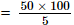= Rs 1000

QUESTION: 18

Find out the term in the number series which is wrong.
3, 10, 27, 4, 16, 64, 5, 25, 125

Solution:
QUESTION: 19

Choose the missing term out of the given alternatives.
shg rif qje pkd ?

Solution:
QUESTION: 20

Choose the missing term out of the given alternatives.
cx fu ir ? ol ri

Solution:
QUESTION: 21

In this letter series, some of the letters are missing. Choose the correct letter given below -
ac _ cab _ baca _ aba _ acac

Solution:

The series  is acac / abab / acac / abab / acac.

QUESTION: 22

Find out the term in the number series which is wrong.
3, 2, 8, 9, 13, 22, 18, 32, 23, 42

Solution:

The given sequence is a combination of two series :
I.   3, 8, 13, 18, 23  and
II   2, 9, 22, 32,42
The pattern in I is + 5 and the pattern in II is + 10.
So, in II, 9 is wrong and must be replaced by (2 + 10)  i.e. 12

QUESTION: 23

762.78 x 3.5 + 112.47 = ? - 1518.94

Solution:

2669.73 + 112.47 = x - 1518.94
⇒ 2782.2 = x - 1518.94
⇒ x = 4301.14

QUESTION: 24

? - 8539.68 = 4628.73

Solution:
QUESTION: 25

A and B together take 5 days to do a work, B and C take 7 days to do the same, and A and C take 4 days to fo it. Who among these will take the least time if put to do it alone?

Solution:

(A + B)'s 1 day's work = 1 5 ; (B + C)'s 1 day's work = 1/7
and (A + C )'s 1 day's work = 1/4
2(A + B + C)'s 1 day's work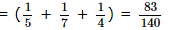∴ (A + B + C)'s 1 day's work = 83/280
A's 1 day's work
= (A + B + C)'s 1 day's work - (B + C)'s 1 day's work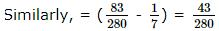B's 1 day's work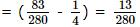C's 1 day's work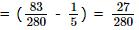Thus time taken by A, B, C is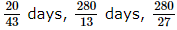days respectively
The time taken by A is least